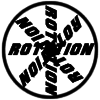#### You may also like### 8 Methods for Three by One

This problem in geometry has been solved in no less than EIGHT ways by a pair of students. How would you solve it? How many of their solutions can you follow? How are they the same or different? Which do you like best?### Rots and Refs

Follow hints using a little coordinate geometry, plane geometry and trig to see how matrices are used to work on transformations of the plane.### Reflect Again

Follow hints to investigate the matrix which gives a reflection of the plane in the line y=tanx. Show that the combination of two reflections in intersecting lines is a rotation.

# Matrix Meaning

### Why do this problem?

This problem asks students to consider the geometrical properties of matrix transformations in order to gain a greater understanding of matrix algebra, in 2 and 3 dimensions.

### Possible approach

The problem works well as a discussion activity. Students could work with a partner and consider each statement first in 2D and then in 3D. After allowing them some time to consider the statements, work with examples, and think about the geometrical interpretation of the situation, bring the class together to discuss their ideas.

Encourage justifications which use geometrical reasoning as well as those using algebra. If a statement is sometimes true, it is important for students to identify when it is true, and geometrically speaking, why there are situations where it is and isn't true.

### Key questions

When you perform two transformations, does the order matter?
M and N are neither reflections nor rotations - what other types of transformation could they represent?

### Possible extension

Construct matrices in three dimensions which make each statement true or not true.

### Possible support

Transformations for 10 offers a chance to think about transformations effected by different matrices.